# Up-sampling with Transposed Convolution

If you’ve heard about the transposed convolution and got confused what it actually means, this article is written for you.

The content of this article is as follows:

The notebook is available in my GitHub.

# The Need for Up-sampling

When we use neural networks to generate images, it usually involves up-sampling from low resolution to high resolution.

There are various methods to conduct up-sampling operation:

All these methods involve some interpolation method which we need to chose when deciding a network architecture. It is like a manual feature engineering and there is nothing that the network can learn about.

# Why Transposed Convolution?

If we want our network to learn how to up-sample optimally, we can use the transposed convolution. It does not use a predefined interpolation method. It has learnable parameters.

It is useful to understand the transposed convolution concept as it is used in important papers and projects such as:

FYI — the transposed convolution is also known as:

We will only use the word transposed convolution in this article but you may notice alternative names in other articles.

# Convolution Operation

Let’s use a simple example to explain how convolution operation works. Suppose we have a 4x4 matrix and apply a convolution operation on it with a 3x3 kernel, with no padding, and with a stride of 1. As shown further below, the output is a 2x2 matrix.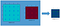Convolution Operation

The convolution operation calculates the sum of the element-wise multiplication between the input matrix and kernel matrix. Since we have no padding and the stride of 1, we can do this only 4 times. Hence, the output matrix is 2x2.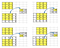Sum of Element-wise Multiplication

One important point of such convolution operation is that the positional connectivity exists between the input values and the output values.

For example, the top left values in the input matrix affect the top left value of the output matrix.

More concretely, the 3x3 kernel is used to connect the 9 values in the input matrix to 1 value in the output matrix. A convolution operation forms a many-to-one relationship. Let’s keep this in mind as we need it later on.

# Going Backward

Now, suppose we want to go the other direction. We want to associate 1 value in a matrix to 9 values in another matrix. It’s a one-to-many relationship. This is like going backward of convolution operation, and it is the core idea of transposed convolution.

For example, we up-sample a 2x2 matrix to a 4x4 matrix. The operation maintains the 1-to-9 relationship.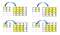Going Backward of Convolution

But how do we perform such operation?

To talk about how, we need to define the convolution matrix and the transposed convolution matrix.

# Convolution Matrix

We can express a convolution operation using a matrix. It is nothing but a kernel matrix rearranged so that we can use a matrix multiplication to conduct convolution operations.

We rearrange the 3x3 kernel into a 4x16 matrix as below:

This is the convolution matrix. Each row defines one convolution operation. If you do not see it, the below diagram may help. Each row of the convolution matrix is just a rearranged kernel matrix with zero padding in different places.

To use it, we flatten the input matrix (4x4) into a column vector (16x1).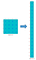Flattened Input Matrix

We can matrix-multiply the 4x16 convolution matrix with the 16x1 input matrix (16 dimensional column vector).

The output 4x1 matrix can be reshaped into a 2x2 matrix which gives us the same result as before.

In short, a convolution matrix is nothing but an rearranged kernel weights, and a convolution operation can be expressed using the convolution matrix.

So what?

The point is that with the convolution matrix, you can go from 16 (4x4) to 4 (2x2) because the convolution matrix is 4x16. Then, if you have a 16x4 matrix, you can go from 4 (2x2) to 16 (4x4).

Confused?

Please read on.

# Transposed Convolution Matrix

We want to go from 4 (2x2) to 16 (4x4). So, we use a 16x4 matrix. But there is one more thing here. We want to maintain the 1 to 9 relationship.

Suppose we transpose the convolution matrix C (4x16) to C.T (16x4). We can matrix-multiply C.T (16x4) with a column vector (4x1) to generate an output matrix (16x1). The transposed matrix connects 1 value to 9 values in the output.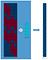Convolution By Matrix Multiplication

The output can be reshaped into 4x4.

We have just up-sampled a smaller matrix (2x2) into a larger one (4x4). The transposed convolution maintains the 1 to 9 relationship because of the way it lays out the weights.

NB: the actual weight values in the matrix does not have to come from the original convolution matrix. What’s important is that the weight layout is transposed from that of the convolution matrix.

# Summary

The transposed convolution operation forms the same connectivity as the normal convolution but in the backward direction.

We can use it to conduct up-sampling. Moreover, the weights in the transposed convolution are learnable. So we do not need a predefined interpolation method.

Even though it is called the transposed convolution, it does not mean that we take some existing convolution matrix and use the transposed version. The main point is that the association between the input and the output is handled in the backward fashion compared with a standard convolution matrix (one-to-many rather than many-to-one association).

As such, the transposed convolution is not a convolution. But we can emulate the transposed convolution using a convolution. We up-sample the input by adding zeros between the values in the input matrix in a way that the direct convolution produces the same effect as the transposed convolution. You may find some article explains the transposed convolution in this way. However, it is less efficient due to the need to add zeros to up-sample the input before the convolution.

One caution: the transposed convolution is the cause of the checkerboard artifacts in generated images. This article recommends an up-sampling operation (i.e., an interpolation method) followed by a convolution operation to reduce such issues. If your main objective is to generate images without such artifacts, it is worth reading the paper to find out more.

# References

##  A guide to convolution arithmetic for deep learning

Vincent Dumoulin, Francesco Visin

https://arxiv.org/abs/1603.07285

##  Unsupervised Representation Learning with Deep Convolutional Generative Adversarial Networks

Alec Radford, Luke Metz, Soumith Chintala

https://arxiv.org/pdf/1511.06434v2.pdf

##  Fully Convolutional Networks for Semantic Segmentation

Jonathan Long, Evan Shelhamer, Trevor Darrell

https://people.eecs.berkeley.edu/~jonlong/long_shelhamer_fcn.pdf

##  Deconvolution and Checkerboard Artifacts

Augustus Odena, Vincent Dumoulin, Chris Olah

https://distill.pub/2016/deconv-checkerboard/

## More from Naoki

https://twitter.com/naokishibuya